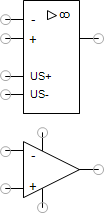# Operational amplifier with power supply pinsThe operational amplifier amplifies the differential input voltage U_in with

U_in = U_n – U_p

by the open-loop voltage gain V0, which inverts the output voltage U_out.

Given an ideal linear model, the output voltage can therefore be calculated with the following equation:

U_out = -Vo * U_in

Additionally, the supply voltage restricts the output voltage.

The connectors of the supply voltage need to be interconnected, in order for the operational amplifier to work.

When the non-linear model is used (which is FluidSIMs default setting), the previously stated equation is only satisfied by approximation. The output voltage approaches the limiting voltage without abrupt change in the slope.

The inputs are provided with a high resistance while the output is provided with a low resistance.

The operational amplifier is not modelled as an integrated circuit in detail. The temporal relation between input and output signal is modelled as a PT1-System with time constant T.